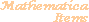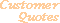Home Older Mathematica Items

Scientific Arts

# Compilation and Packed Arrays

## An Example of the Use of Compile in a Procedural Program: The Mandelbrot Set

Here is procedural program that generates the famous Mandelbrot set (this particular piece of code has appeared in many sources).

``````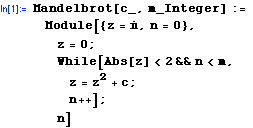``````

This is a compiled version.

``````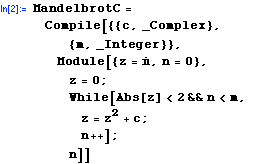```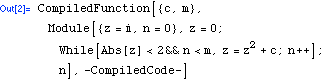```

The output above is displayed in an abbreviated form. You can see the internal representation of the CompiledFunction object by using the function InputForm.

``````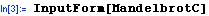``````
```CompiledFunction[{_Complex, _Integer}, {{4, 0, 0}, {2, 0, 0}},  {4, 5, 4, 6, 0}, {{1, 3}, {11, 0. + 1.*I, 1}, {9, 0, 1}, {9, 0, 2},   {19, 0, 2, 0}, {10, 0., 1}, {20, 0, 1, 2}, {16, 2, 1},   {95, 36, 4, 0, 1, 3, 0, 2}, {9, 2, 2}, {19, 0, 2, 3}, {56, 2, 3, 0},   {48, 0, 4}, {55, 1, 0, 1}, {13, 1, 3}, {49, 3}, {8, False, 2}, {13, 2, 3},   {48, 3, 10}, {16, 1, 3}, {35, 3, 3, 4}, {31, 4, 0, 5}, {16, 5, 1},   {14, 1, 3}, {9, 1, 4}, {29, 1, 4, 4}, {14, 4, 1}, {49, -19}, {3, 1}},  Function[{c, m}, Module[{z = I, n = 0},    z = 0; While[Abs[z] < 2 && n < m, z = z^2 + c; n++]; n]]]
```

The compiled function contains the opcode instructions for the "virtual machine" while still retaining the full Mathematica code for use when the arguments are not of the type expected by Compile (in this case that the first argument be complex and the second argument be an integer).

This computes the Mandelbrot function on a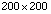grid of 40000 points using the complied version of the function. The plot output is suppressed through the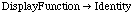option setting, but we give it the name MandelPlot so that we can display it later.

``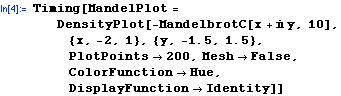``
```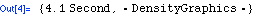```

Here is the timing comparison for then non-compiled version (on a Macintosh Powerbook 333 MHz G3).

``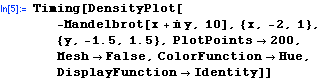``
```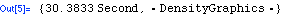```

We see that there is a roughly a factor of 7.4 increase in time relative to the compiled version.

Here is the graphic:

``````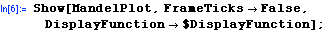``````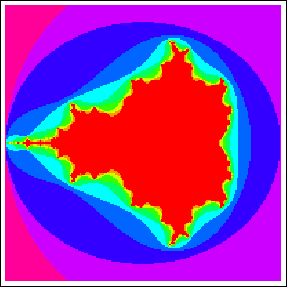## Packed Arrays

It should also be noted that, because of the new PackedArray technology in Mathematica 4, there are many automatic speedups when doing calculations with arrays where the array elements are of a uniform type.

Packed arrays provide an alternative format for vectors, matrices, and tensors of machine numbers, (integers, reals and complex reals). This alternative format coexists with the traditional format for arrays that has always existed in Mathematica. The packed and the unpacked formats both behave in equivalent ways, the system converts from one to the other as necessary.

Some of the benefits of packed arrays are that they use less memory and that many computations can be faster.

Here we define a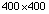matrix populated with random numbers between 0 and 1.

``````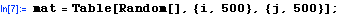``````

The Developer` context contains functions that allow you to see whether or not an array is a PackedArray.

``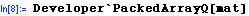``
```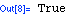```

Many of Mathematica's functions have been optimized to act very efficiently on arrays that have a uniform type.

``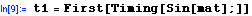``
``````

Now let's change one element of the array.

``````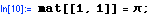``````

Since the first element is now an exact number the array is no longer a packed array.

``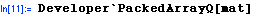``
```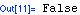```

As a consequence the execution time is close to a factor of 9 greater.

``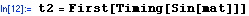``
```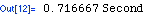```
``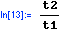``
```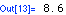```

There is much more that can be said about packed arrays. This only scratches the surface.

 For further information on our services send email to info@scientificarts.com . Contents of this web site Copyright © 1999-2011, Scientific Arts, LLC.
 s